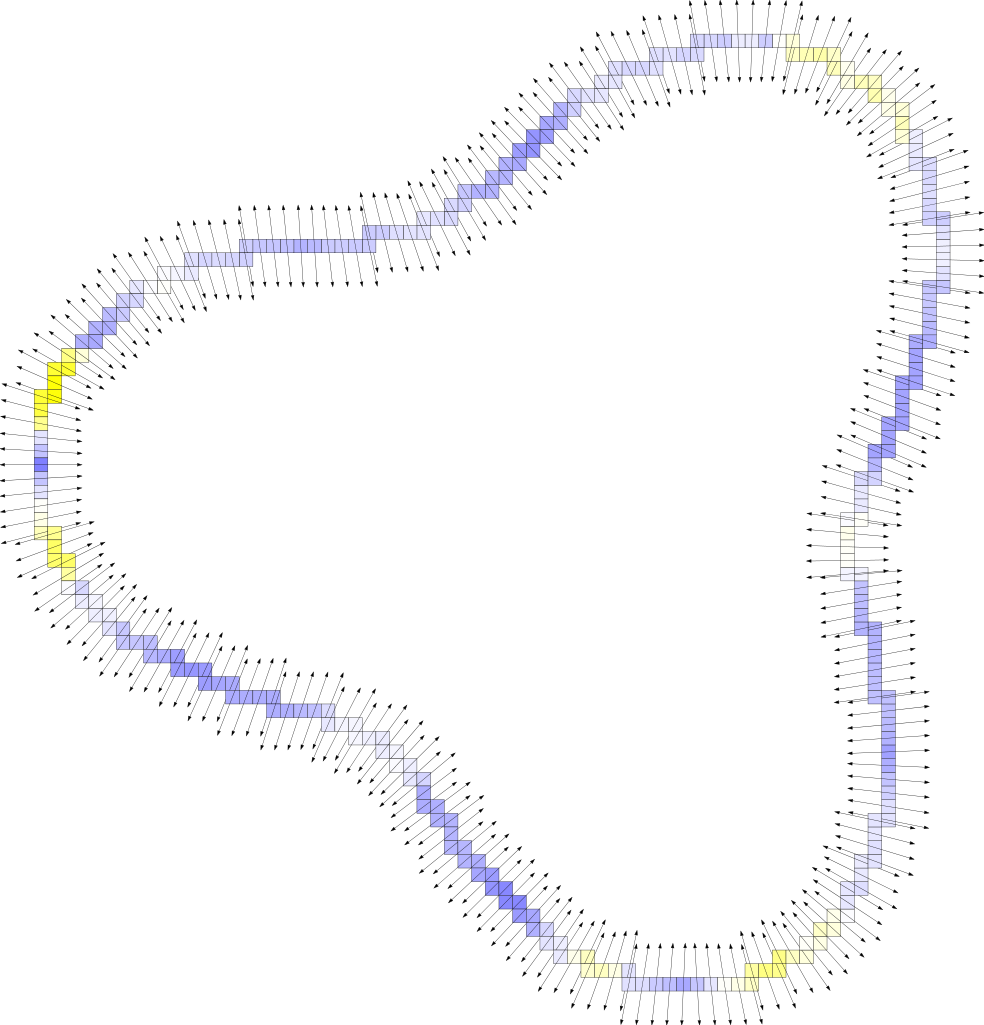DGtal  1.3.beta
geometry/volumes/dvcm-2d.cpp

This example shows the computation of the VCM of a set of 2D digital points. The normal is estimated from the diagonalization of the VCM tensor. Feature detection is achieved with the eigenvalues of the VCM. A red color indicates a feature. Normals are displayed as arrows.

Computing the Voronoi Covariance Measure of a point set
\$ ./examples/geometry/volumes/dvcm-2dNormal vector and feature detection with Voronoi Covariance Measure.
#include <iostream>
#include "DGtal/base/Common.h"
#include "DGtal/helpers/StdDefs.h"
#include "DGtal/kernel/BasicPointPredicates.h"
#include "DGtal/math/linalg/EigenDecomposition.h"
#include "DGtal/geometry/volumes/distance/ExactPredicateLpSeparableMetric.h"
#include "DGtal/geometry/volumes/estimation/VoronoiCovarianceMeasure.h"
#include "DGtal/io/boards/Board2D.h"
#include "ConfigExamples.h"
using namespace std;
using namespace DGtal;
int main( int /* argc */, char** /* argv */ )
{
typedef Z2i::Space Space;
typedef Z2i::Point Point;
typedef ExactPredicateLpSeparableMetric<Space, 2> Metric; // L2-metric
typedef EigenDecomposition<2,double> LinearAlgebraTool;
typedef LinearAlgebraTool::Matrix Matrix;
// Gets the points
vector<unsigned int> vPos;
vPos.push_back(0);
vPos.push_back(1);
string inputSDP = examplesPath + "samples/flower-30-8-3.sdp";
// string inputSDP = examplesPath + "samples/ellipse-20-7-0.4.sdp";
// string inputSDP = examplesPath + "samples/accflower-20-5-5-0.1.sdp";
trace.info() << "Reading input 2d discrete points file: " << inputSDP;
trace.info() << " [done] " << std::endl ;
const double R = 20;
trace.info() << "Big radius R = " << R << std::endl;
const double r = 5;
trace.info() << "Small radius r = " << r << std::endl;
const double T = 0.1;
trace.info() << "Feature thres. T = " << T << std::endl; // threshold for displaying features as red.
const double size = 3.0; // size of displayed normals
Metric l2;
VCM vcm( R, ceil( r ), l2, true );
vcm.init( pts.begin(), pts.end() );
Domain domain = vcm.domain();
KernelFunction chi( 1.0, r );
// Flat zones are metallic blue, slightly curved zones are white,
// more curved zones are yellow till red.
colormap.addColor( Color( 128, 128, 255 ) );
colormap.addColor( Color( 255, 255, 255 ) );
colormap.addColor( Color( 255, 255, 0 ) );
colormap.addColor( Color( 255, 0, 0 ) );
Board2D board;
Matrix vcm_r, evec;
RealVector eval;
for ( std::vector<Point>::const_iterator it = pts.begin(), itE = pts.end();
it != itE; ++it )
{
// Compute VCM and diagonalize it.
vcm_r = vcm.measure( chi, *it );
LinearAlgebraTool::getEigenDecomposition( vcm_r, evec, eval );
double feature = eval[ 0 ] / ( eval[ 0 ] + eval[ 1 ] );
board << CustomStyle( it->className(),
new CustomColors( Color::Black, colormap( feature > T ? T : feature ) ) )
<< *it;
// Display normal
RealVector normal = evec.column( 1 );
RealPoint p( (*it)[ 0 ], (*it)[ 1 ] );
Display2DFactory::draw( board, size*normal, p );
Display2DFactory::draw( board, -size*normal, p );
}
board.saveSVG("dvcm-hat-r.svg");
return 0;
}
// //
DGtal::HyperRectDomain< Space >
DGtal::Color
Structure representing an RGB triple with alpha component.
Definition: Color.h:67
DGtal::EigenDecomposition
Aim: This class provides methods to compute the eigen decomposition of a matrix. Its objective is to ...
Definition: EigenDecomposition.h:86
DGtal::trace
Trace trace
Definition: Common.h:154
Aim: Implements method to read a set of points represented in each line of a file.
DGtal::SpaceND< 2, Integer >
DGtal::CustomStyle
Definition: Board2D.h:217
DGtal::Trace::info
std::ostream & info()
draw
void draw(const Iterator &itb, const Iterator &ite, Board &aBoard)
Definition: testSegmentation.cpp:75
DGtal
DGtal is the top-level namespace which contains all DGtal functions and types.
Domain
HyperRectDomain< Space > Domain
Definition: testSimpleRandomAccessRangeFromPoint.cpp:44
DGtal::CustomColors
Custom style class redefining the pen color and the fill color. You may use Board2D::Color::None for ...
Definition: Board2D.h:278
LibBoard::Board::saveSVG
void saveSVG(const char *filename, PageSize size=Board::BoundingBox, double margin=10.0) const
Definition: Board.cpp:1012
DGtal::VoronoiCovarianceMeasure< Space, Metric >
main
int main(int argc, char **argv)
Definition: testArithmeticDSS-benchmark.cpp:147
DGtal::Board2D
Aim: This class specializes a 'Board' class so as to display DGtal objects more naturally (with <<)....
Definition: Board2D.h:70
RealVector
Space::RealVector RealVector
Definition: fullConvexitySphereGeodesics.cpp:113
DGtal::ExactPredicateLpSeparableMetric
Aim: implements separable l_p metrics with exact predicates.
Definition: ExactPredicateLpSeparableMetric.h:87
domain
Domain domain
Definition: testProjection.cpp:88
DGtal::PointVector< dim, Integer >
Space
SpaceND< 2 > Space
Definition: testSimpleRandomAccessRangeFromPoint.cpp:42
Point
MyPointD Point
Definition: testClone2.cpp:383
RealPoint
Z2i::RealPoint RealPoint
Definition: testAstroid2D.cpp:46